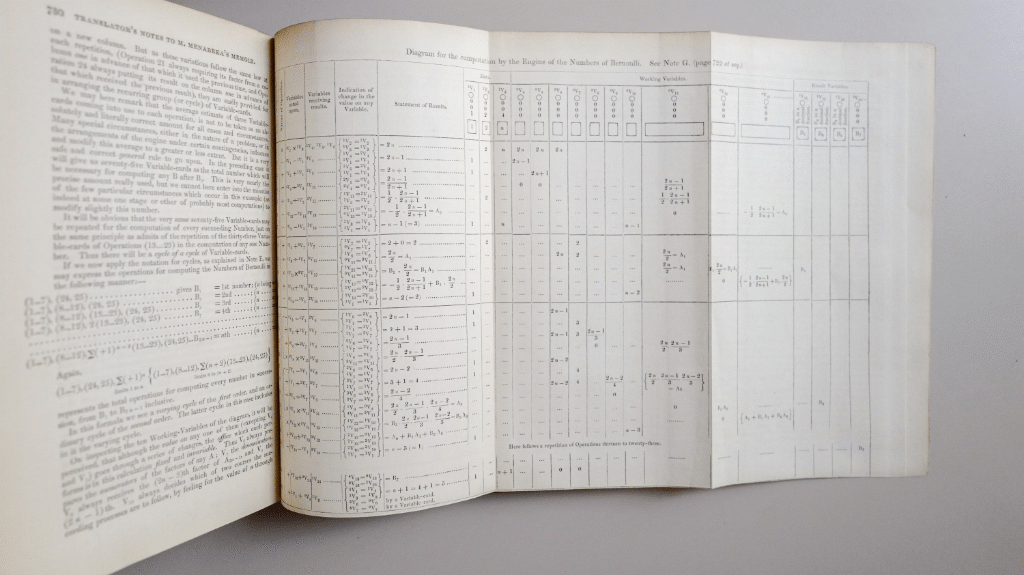Ada Lovelace’s ‘Notes’ contained the first published computer program. More of a trace than an actual listing, it used an iterative algorithm to calculate Bernoulli numbers.Here is the recreated algorithm in Julia. Ada’s original didn’t describe conditions for the repetition of operations 13 to 23, and it wasn’t clear how the array of result variables would actually be populated on the Analytical Engine. However, everything else is pretty much how her program was originally described (apart from fixing Ada’s bug in operations 4 and 24).

The results, converting to current notation (Ada’s program calculates B2n), are:

B2 = 1/6
B4 = -1/30
B6 = 1/42
B8 = -1/30
B10 = 5/66
B12 = -691/2730
B14 = 7/6
B16 = -3617/510
B18 = 43867/798
B20 = -174611/330